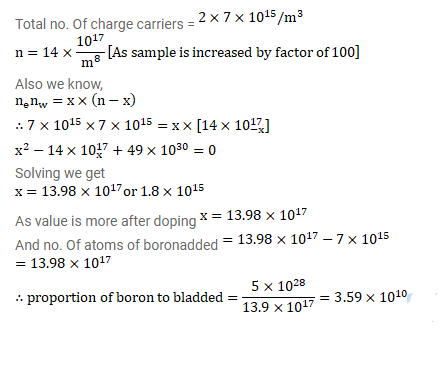# Estimate the proportion of boron impurity which will increase the conductivity

Question:

Estimate the proportion of boron impurity which will increase the conductivity of a pure silicon sample by a factor of $100 .$ Assume that each boron atom creates a hole and the concentration of holes in pure silicon at the same temperature is $7 \times 10^{16}$ holes per cubic metre. Density of silicon is $5 \times 10^{28}$ atoms per cubic metre.

Solution: